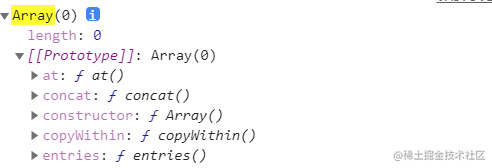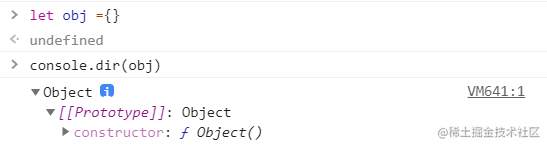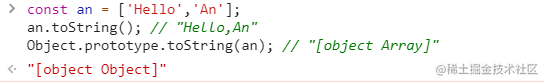判断数组的方法（面试问到的，下一篇我会写一篇关于跨域的文章）

废话不多刷！直接来正题吧！

1.使用数组自带的方法：Array.isArray() 常用方法

let arr=[1,2,3]
let obj={}
console.log(Array.isArray(arr)) //true
console.log(Array.isArray(obj)) //false

2.使用constructor(constructor是一个构造器，指明了是由什么构造出来的) 其实 let arr=[] 可以这样写

let arr= new Array()

console.log(arr) //有一个constructor:f Array()let arr=[1,2,3]
let obj={}
console.log(arr.constructor===Array) //true
console.log(obj.constructor==Array) //false

3.使用instanceof()instanceof 运算符用于检测构造函数的 prototype 属性是否出现在某个实例对象的原型链

let arr=[1,2,3]
let obj={}
console.log(arr instanceof Array) //true
console.log(obj instanceof Array) //false

4.使用isprototypeof()(该方法用于判断一个对象是否存在一个对象的原型链上)

let arr = [];//上面提过相当于let arr =new Array()
console.log(Array.prototype.isPrototypeOf(arr)); // true

5.跨原型链调用tostring()const an = ['Hello','An'];
an.toString(); // "Hello,An"
Object.prototype.toString.call(an); // "[object Array]"